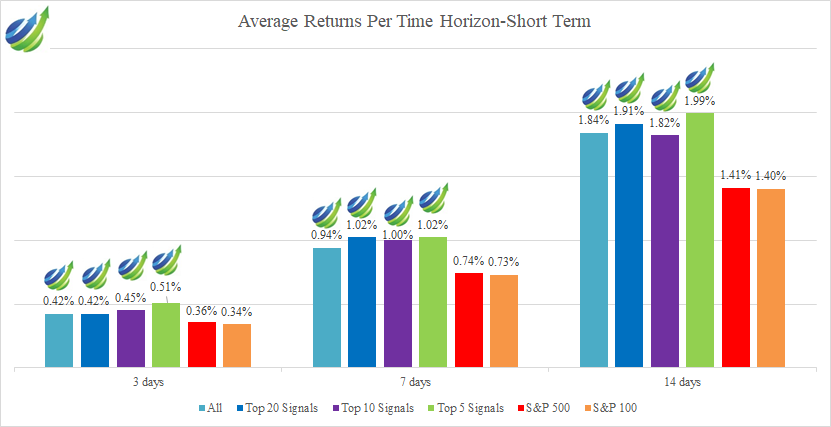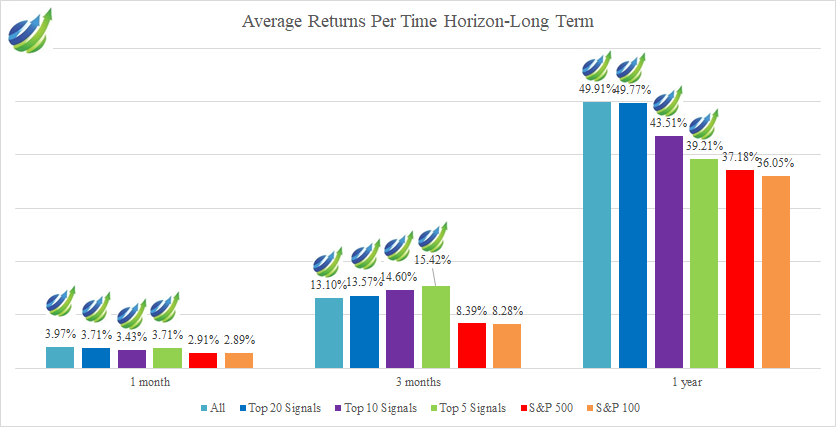# S&P 100 Stocks: AI Outperforms S&P 500 by 12.59% with an Accuracy of 99%

## Executive Summary

The purpose of this forecast report is to present the results of the live forecast performance evaluation for the S&P 100 Stocks Package by the I Know First AI Algorithm. The following results were observed when signal and predictability filters were applied to pick the best-performing stocks out of the most predictable ones. The evaluation period is from 30th April 2020 to 29th August 2021. The corresponding returns distribution of stock signal filters for this package is shown below:

## The S&P 100 Stock Package Highlights:

• The highest average return is 49.91% for the All Signals on a 1-year time horizon
• Predictions reach up to 99% hit ratio regardless of economic conditions amid COVID-19
• The S&P 500 benchmark has an overall increasing trend and has gained by 53.41% over the evaluation period from 30th April 2020 to 29th August 2021. The S&P 100 has a return of 54.04% for the same period of time
• Even during the pandemic, all the stocks forecast have outperformed the S&P 500 and the S&P 100 indexes
• I Know First provides an investment strategy for institutional investors that has generated a return of 71.43% and has exceeded the S&P 500 return by 18.02% for the analized period

## About the I Know First Algorithm

The I Know First self-learning algorithm analyzes, models, and predicts the stock market. The algorithm is based on Artificial Intelligence (AI) and Machine Learning (ML) and incorporates elements of Artificial Neural Networks and Genetic Algorithms.

The system outputs the predicted trend as a number, positive or negative, along with a wave chart that predicts how the waves will overlap the trend. This helps the trader to decide which direction to trade, at what point to enter the trade, and when to exit. Since the model is 100% empirical, the results are based only on factual data, thereby avoiding any biases or emotions that may accompany human derived assumptions.

The human factor is only involved in building the mathematical framework and providing the initial set of inputs and outputs to the system. The algorithm produces a forecast with a signal and a predictability indicator. The signal is the number in the middle of the box. The predictability is the number at the bottom of the box. At the top, a specific asset is identified. This format is consistent across all predictions.

Our algorithm provides two independent indicators for each asset – Signal and Predictability.

The Signal is the predicted strength and direction of the movement of the asset. Measured from -inf to +inf.

The predictability indicates our confidence in that result. It is a Pearson correlation coefficient between past algorithmic performance and actual market movement. Measured from -1 to 1.

You can find a detailed description of our heatmap here.

## The Stock Market Forecast Performance Evaluation Method

We perform evaluations on the individual forecast level. It means that we calculate what would be the return of each forecast we have issued for each horizon in the testing period. Then, we take the average of those results by strategy and forecast horizon.

For example, to evaluate the performance of our 1-month forecasts, we calculate the return of each trade by using this formula:

This simulates a client purchasing the asset based on our prediction and selling it exactly 1 month in the future.

We iterate this calculation for all trading days in the analyzed period and average the results.

Note that this evaluation does not take a set portfolio and follow it. This is a different evaluation method at the individual forecast level.

## The Hit Ratio Method

The hit ratio helps us to identify the accuracy of our algorithm’s predictions.

Using our Daily Forecast asset filtering, we predict the direction of the movement of different assets. Our predictions are then compared against the actual movements of these assets within the same time horizon.

The hit ratio is then calculated as follows:

For instance, a 90% hit ratio for a predictability filter with a top 10 signal filter would imply that the algorithm correctly predicted the price movements of 9 out of 10 assets within this particular set of assets.

## The Benchmarking Method – S&P 500 Index

In order to evaluate our algorithm’s performance in comparison to the US market, we used the S&P 500 index as a benchmark.

The S&P 500 measures the stock performance of the largest 500 companies by market cap listed on different stock exchanges in the United States. It is one of the most followed equity indices and is frequently used as the best indicator for the overall performance of US public companies, and the US market as a whole. The S&P 500 is a capitalization-weighted index, the weight of each company in the index is determined based on its market cap divided by the aggregate market cap of all the S&P 500 companies.

For each time horizon, we compare the S&P 500 performance with the performance of our forecasts.

## S&P 100 Stocks Package Performance Evaluation – Overview

In this report, we conduct testing for S&P 100 stocks that I Know First covers by its algorithmic forecast. The period for evaluation and testing is from April 30th, 2020 to August 29th, 2021. During this period, we were providing our clients with daily forecasts in time horizons spanning from 3 days to 1 year which we evaluate in this report.(Table 1: Average Returns Per Time Horizon for the S&P 100 Stocks Package)

As can be seen in the table above, our algorithm provided positive returns for most of the time horizons. The S&P 500 benchmark was outperformed in most signal groups for most of the time horizons. We found that by using the signal indicators as selection criteria for filtering for the best stock picks we had greater returns on all forecasting horizons. Most notably, we saw the highest return for the 1-year horizon at 49.91% which greatly exceeds the S&P 500 benchmark return of 37.18% by 12.73%.(Figure 1: Average Returns Per Time Horizon Short Term for the S&P 100 Stocks Package)(Figure 2: Average Returns Per Time Horizon Long Term for the S&P 100 Stocks Package)

From the above charts, it is evident that as the forecasting horizon expands, the average returns tend to become higher. Above all, the returns for the longer horizons provided premiums above the S&P 500 average by a larger percentage comparably to the shorter-term horizons in the same signal group. Ultimately, the I Know First algorithm shows the highest average return for the 1-year forecast as 49.91% for the All Signals which exceeds the S&P 500 index by 12.73%. In the 14-day period, the AI Algorithm was able to generate the highest return of 1.99% for the Top 5 Signals which exceeds the S&P 500 index by 0.58%.(Table 2: Hit Ratio Per Time Horizon for the S&P 100 Stocks Package)

According to the table above, all the signal groups across all time horizons gave a hit ratio greater than 54%. It should be noted that as the time horizon gets longer, I Know First hit ratios gradually increase from the 54% ratio interval for the short-term horizons to 99% at the Top 20 Signals and Top 5 Signal subsets for 1 year. All across the board, as the time horizon gets longer the hit ratio increases for the All Signal indicators by 43%, Top 20 by 44% Top 10 by 43%, and 5 Signals by 45%. Examining Table 2 shows that a hit ratio for All Signals is associated with a high average return, and as the forecast range expands, the return increases in performance.(Figure 3: Hit Ratio Per Time Horizon of the S&P 100 Stocks Package)

Looking at Figure 3, it is clear that at the short term horizons the hit ratios are relatively low at all signal indicators but the hit ratios increase over the long term horizons showing the I Know First Algorithm is able to successfully predict most of the stock movements.

I Know First has used algorithmic outputs from the S&P 100 Stocks package to provide investment strategies for institutional investors.(Figure 4: I Know First trading result for the period from April 30, 2020, to August 29, 2021)

The investment strategy that was recommended to institutional investors by I Know First has accumulated a return of 71.43% that has exceeded the S&P 500 return by 18.02%. Moreover, we can notice that the I Know First cumulative return is consistently higher than the S&P 500 return for the whole analyzed period.

## S&P 100 Stocks Package Forecast: Conclusion

This report looked at the live performance forecast of I Know First data for S&P 100 Stocks Package from April 30, 2020 to August 29, 2021. From the above data, we can observe that the I Know First Algorithm is exceeding the S&P 500 benchmark index across all signal filtering subsets and forecasting periods. Data from Figures 1 and 2 above shows I Know First was able to generate a return that exceeded the S&P 500 return by 12% in one year. In the 14-day period, the Top 5 Signal index is 1.99% that exceeds the S&P500 index by 0.58%. Moreover, I Know First has used AI outputs to provide an investment strategy for institutional investors that has generated a return of 71.43% and has exceeded the S&P 500 return by 18.02% for the analyzed period.